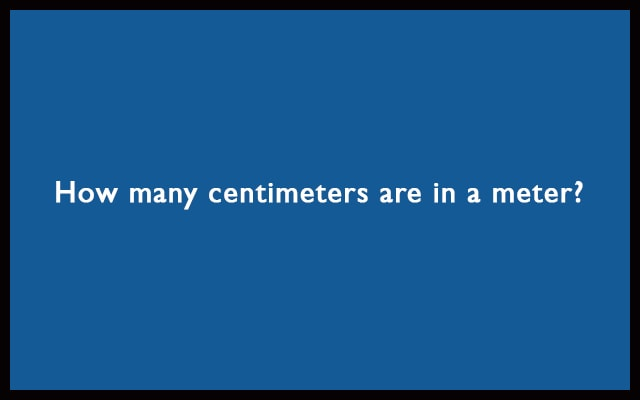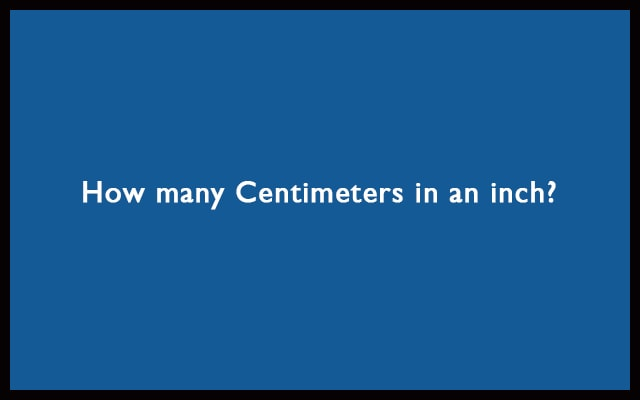# Length## How many centimeters are in a meter? – m to cm conversion

Hello friends, are you looking for the answer to How many centimeters are in a meter? Here you will get the relation between meters and cm with some examples. As we know, 1 meter is equal to 100 centimeters. So, the relation between meter and centimeter is: 1 m = 100 cm How many centimeters …## How many Centimeters in an inch? – Inches to cm Conversion

Hello visitors, are you looking for the answer to How many Centimeters in an Inch? Here you will get the relation between inches and cm with some examples. As we know, 1 inch is equal to 2.54 centimeters. So, the relation between inches and centimeter is: 1 inch = 2.54 cm How many Centimeters in …